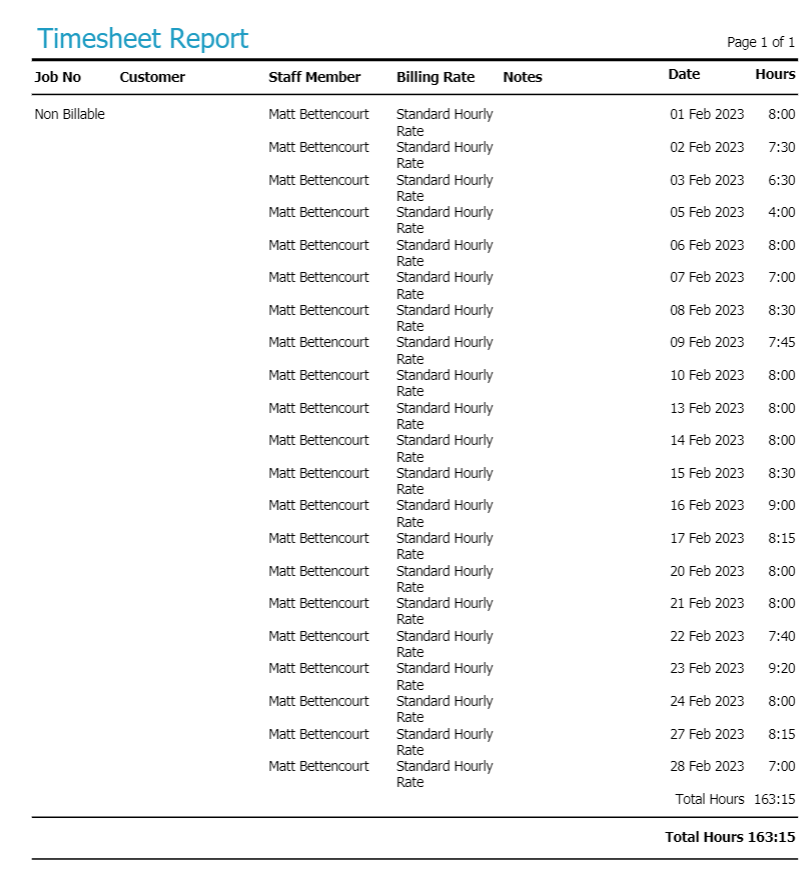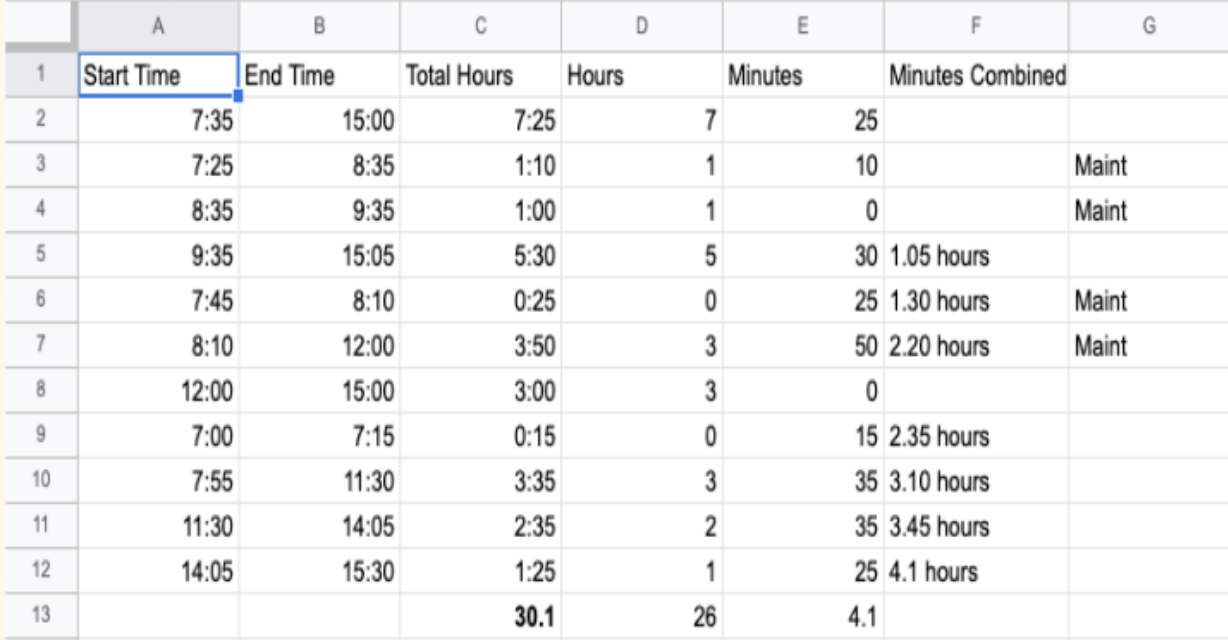Many people using Tradify's timesheet report might simply copy and paste the information to a spreadsheet and attempt to add up the hours and minutes manually. However, this can lead to inaccurate calculations as it doesn't account for the fact that 60 minutes equals 1 hour. In this article, we'll show you how to correctly add up timesheet times from a timesheet report in Tradify.

## Example 1:

Let's take Matt's timesheets as an example:• If you add up all the hours (ignoring the minutes), it comes to 159 hours.
• Then, add up the minutes: 30+30+30+30 = 2 hours; 45+15 = 1 hour; 40+20+15 = 1 hour 15 minutes.
• In total, the timesheet comes to 163 hours and 15 minutes.

## Example 2:

Here's another example:• Add up all the hours: 26 hours.
• Add up the minutes: 15+30+45+30 = 2 hours 30 minutes; 45+15+30 = 1 hour 30 minutes.
• In total, the timesheet comes to 30 hours.

By following this method, you can accurately add up timesheet times from a timesheet report in Tradify. This ensures that you are correctly tracking employee hours and facilitating accurate payroll processes.